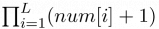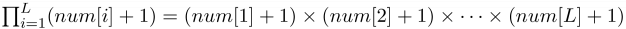## Day 2, Problem 1 - Zoo

Recently, the zookeeper noticed that number of all-eat and no-work animals have been increasing. Take penguins for example – they only care about getting food from the zoo's visitors through showing off their cuteness alone. To fix the bad vibe around the zoo and to ensure that animals only get food via exhibiting their true talents, the zookeeper has decided to set up an algorithms class to teach algorithms to the animals.

One day, the zookeeper decided to lecture the KMP algorithm to the animals.

Zookeeper: "For a given string S of length L, we can construct an array next[] in running time O(L). Can anybody guess the meaning of next[]?

Panda: "Within the first i characters of string S, the length of the longest substring (excluding itself) that is both its prefix and suffix will be stored in next[i]."

Zookeeper: "Very good! Can you give an example?"

Panda: "If S = abcababc, then next = 2. This is because the first 5 characters is abcab, and ab is both its prefix and suffix, and a longer substring with this property does not exist. Likewise, we can find that next = next = next = 0, next = next = 1, next = 2, and next = 3."

The zookeeper commended Panda's studiousness. Then, he carefully explained how next[] could be computed in O(L).

Before dismissing the class, the zookeeper posed a problem: "The KMP algorithm can only generate the array next[]. We wish to obtain an even more powerful num[] array – within the first i characters of string S, the number of substrings that is both its prefix and suffix, and that the prefix and suffix does not overlap, will be stored in num[i]. For example, if S = aaaaa, then num = 2. This is because for the first 4 characters of S, aaaa, substrings a and aa each satisfy the conditions of 'being both a prefix and suffix,' as well as guaranteeing that the prefix and suffix do not overlap. Although substring aaa does satisfy the condition of 'being both a prefix and suffix,' we cannot count it because as simultaneously a prefix and suffix of aaaa, it overlaps with itself. By the same reasoning, we can see that num = 0, num = num = 1, and num = 2."

Finally, the zookeeper designated a prize. The first student to solve this will be rewarded a box of chocolates. After hearing this, Penguin, who has been sleeping for the entire class, has immediately awoken! But Penguin doesn't have a clue how to solve this problem. So, he has scoured the entire zoo to find you for help. Can you help Penguin write a program to compute the num[] array?

Specifically, to avoid huge amounts of output, you do not have to output each value of num[i]. Instead, you will only have to output$\textstyle \prod_{i=1}^{L}(num[i] + 1)$ modulo 1,000,000,007. Here,$\textstyle \prod_{i=1}^{L}(num[i] + 1) = (num + 1) \times (num + 1) \times \cdots \times (num[L] + 1)$.

### Input Format

The first line of input contains a single integer n, representing the number of test cases.
For the next n lines, each line will describe one instance of the problem. Each instance will consist of the single string S. It is guaranteed that S will only consist of lowercase letters.
The input will not contain extra or trailing spaces.

### Output Format

The output should contain n lines, with each line being the answer to the corresponding input string. The order of the answers should be the same as the order of the strings in the input. For each string, the answer should be a single integer representing the answer modulo 1,000,000,007.

### Sample Input

3
aaaaa
ab
abcababc


### Sample Output

36
1
32


### Constraints

The constraints of all the test cases are outlined below.

Test CaseConstraints
1n ≤ 5, L ≤ 50
2n ≤ 5, L ≤ 200
3n ≤ 5, L ≤ 200
4n ≤ 5, L ≤ 10,000
5n ≤ 5, L ≤ 10,000
6n ≤ 5, L ≤ 100,000
7n ≤ 5, L ≤ 200,000
8n ≤ 5, L ≤ 500,000
9n ≤ 5, L ≤ 1,000,000
10n ≤ 5, L ≤ 1,000,000

Point Value: 15 (partial)
Time Limit: 1.00s
Memory Limit: 512M